Score some points with these basketball maths worksheets, covering addition, subtraction, multiplication, and division.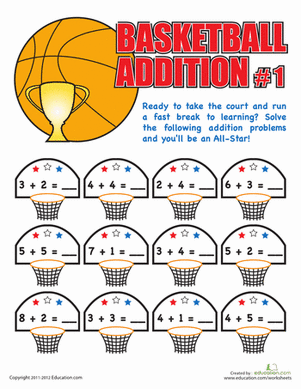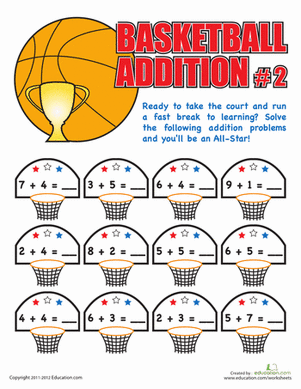Here's a supplemental maths activity that beats boring textbook maths. Your child will work on his addition facts, practising to become an addition all star!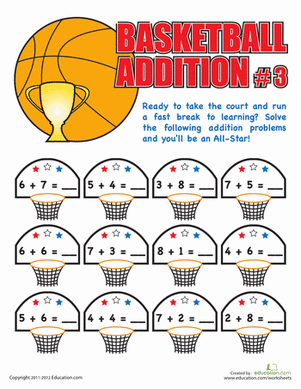How fast can your child do his addition facts? Test his maths skills with a fun basketball maths sheet, and he'll practise doing one-digit addition equations.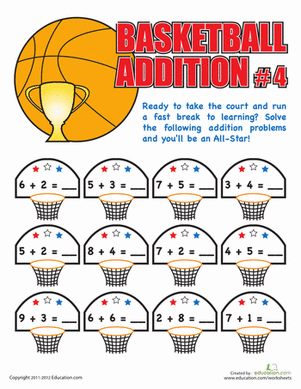Is your child an addition all star? Test his maths handling skills with a fun basketball maths sheet, where he'll work on one-digit addition facts.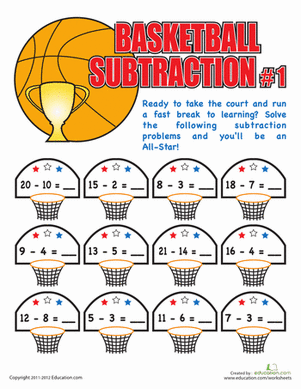Bring your a-game to this subtraction worksheet, and see how many subtraction problems you can solve!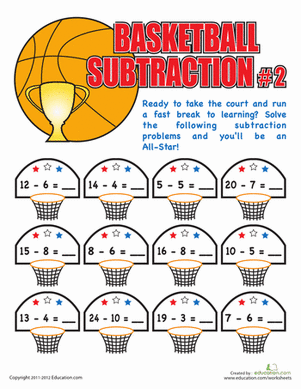How many subtraction shots can you make? Challenge your first grader's subtraction skills with a fun basketball themed maths sheet.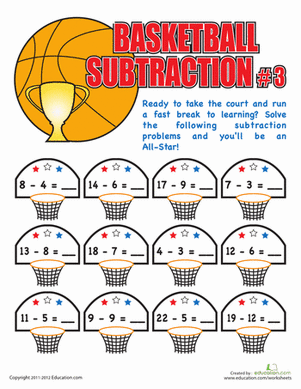If your child is a maths all-star, give him a fun supplemental sheet where he'll get to show off his subtraction skills!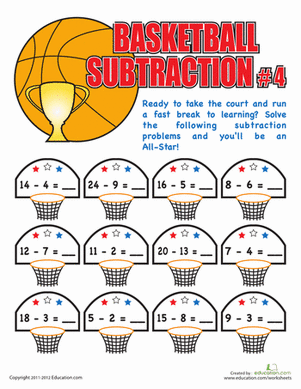See how savvy your child's subtraction skills are with a fun basketball maths sheet. She'll get to do a few one and two digit subtraction problems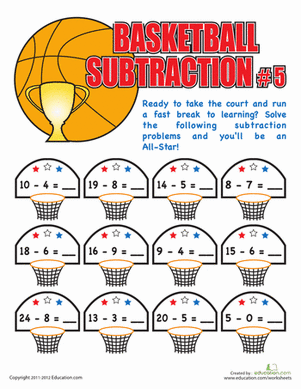Give your all-star maths student a fun challenge with this basketball subtraction sheet! She'll work on one and two digit subtraction problems.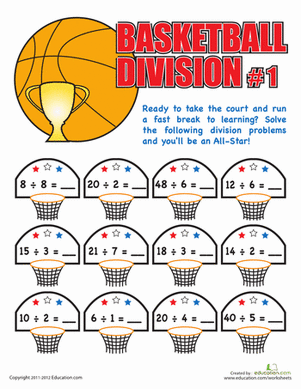Is your child a times tables all-star? Give her a fun challenge with a few division problems, a great way to review the times tables.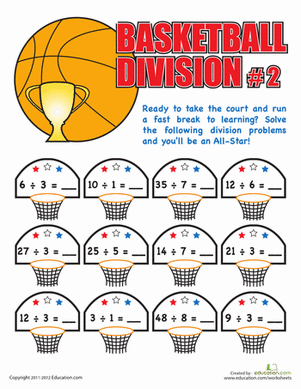Put your child's maths skills to the test with a fun division worksheet. He'll practise some simple division problems, a great way to review times tables!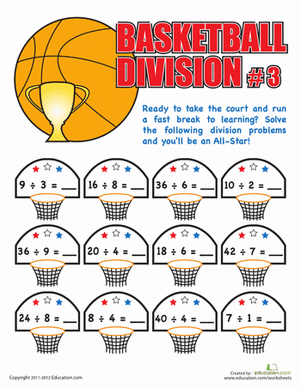Take a break from boring textbook maths, and practise division with a basketball maths sheet! Your child will work on simple division.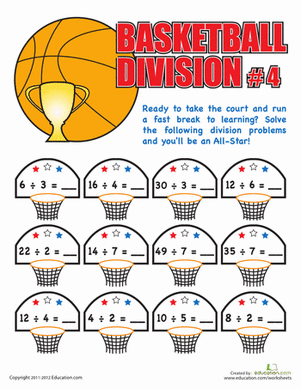Get your child pumped up for maths practise with a fun basketball division sheet! He'll try to complete all the division equations as quick as possible.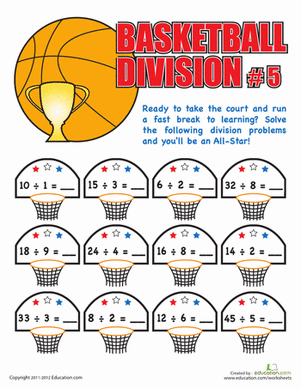Is your maths whiz acing his times tables? Give him a great challenge with this basketball division sheet, where he'll practise division facts.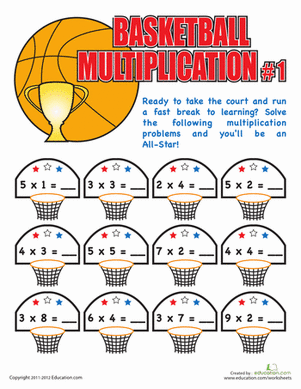Run circles around maths practise with a fun multiplication sheet! Your maths whiz can work on her times tables, basketball style.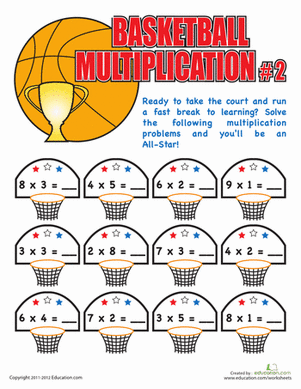Think your child's got a handle on her times tables? Give her a fun challenge with this basketball multiplication sheet.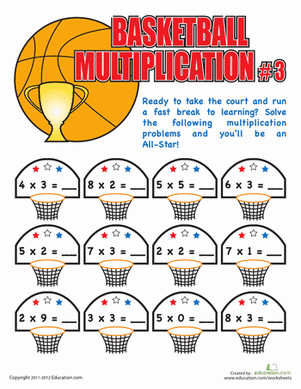Give your all star maths student a fun challenge with this basketball maths sheet! She'll get to review her times tables, perfect practise for a quiz.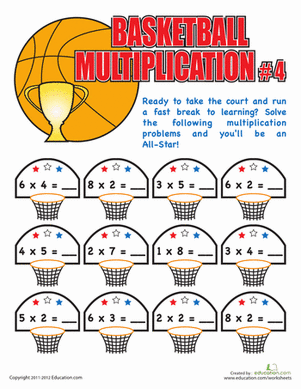Maths maniacs, sneak in some multiplication practise with a fun basketball maths sheet. Your child will get a great review of times tables.

Create new collection

0

### New Collection>

0Items

What could we do to improve Education.com?